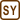## Uknown Gas

### Task number: 1282

The average kinetic energy of a monatomic gas of the amount of substance 1 mol is 2.5 kJ. If we raise the temperature by 300 K, the most probable velocity of the gas molecules will be 642 ms-1. Determine this gas and determine its initial temperature.

• #### Hint – determination of initial temperature

To determine the initial temperature T1 of the unknown gas, we will use the formula for the total kinetic energy of one mole of gas $$\bar{E}_\mathrm{k}$$.

• #### Hint – determination of the gas

To determine the gas, you need to determine its molar mass Mm. To do this, you can use the relation for the most probable velocity vp of gas molecules.

• #### Numerical values

 $$\bar{E}_\mathrm{k}=2.5\,\mathrm{kJ}=2500\,\mathrm{J}$$ the total kinetic energy of one mole of monatomic gas n = 1 mole the amount of substance of the monatomic gas ΔT = 300 K the temperature difference vp = 642 ms−1 the most probable velocity of the gas molecules T1 = ? the initial temperature

From The Handbook of Chemistry and Physics:

 R = 8.31 Jmol−1K−1 the molar gas constant
• #### Analysis

We will determine the initial temperature from the formula decribing the total kinetic energy of one mole of gas, from which we can see that the energy is directly proportional to the thermodynamic temperature.

To determine the gas we will evaluate the molar mass from the relation for the most probable velocity of gas molecules. Then we will compare the value with the values listed in The Handbook of Chemistry and Physics.

• #### Solution

The total kinetic energy of one mole $$\bar{E}_\mathrm{k}$$ of any gas relates with the thermodynamic temperature T through this equation

$\bar{E}_\mathrm{k}=\frac{3}{2}nRT,$

where n is the amount of the substance of the gas and R is the molar gas constant.

This allows us to conduct a direct evaluation of the initial temperature T1 of the unknown gas from the given values. It is true that:

$T_{1}=\frac{2\bar{E}_\mathrm{k}}{3nR}.$

The most probable velocity vp of an ideal gas molecules is given by the relation

$v_\mathrm{p}=\sqrt{\frac{2RT_{2}}{M_m}},$

where Mm is the molar mass of the unknown gas and T2 is the temperature after the gas is heated.

Thereof we can directly express the molar mass of the unknown gas

$M_{\mathrm{m}}=\frac{2RT_{2}}{v^{2}_{\mathrm{p}}}.$

If we substitute the expression

$T_\mathrm{2}=T_\mathrm{1}+\mathrm{\Delta}T$

for the temperature T2, where ΔT is the temperature difference, we obtain

$M_\mathrm{m}=\frac{2R(T_{\mathrm{1}}+\Delta T)}{v^{2}_{\mathrm{p}}}.$
• #### Numerical solution

$T_{1}=\frac{2\bar{E}_\mathrm{k}}{3nR}=\frac{2\cdot{2500}}{3\cdot{1}\cdot{8.31}}\,\mathrm{K}\dot{=}200\,\mathrm{K}$

$M_\mathrm{m}=\frac{2R(T_{\mathrm{1}}+\Delta T)}{v^{2}_{\mathrm{p}}}=\frac{2\cdot{8.31}\cdot(200+300)}{642^{2}}\,\mathrm{kg\,mol}^{-1}$ $M_\mathrm{m}\dot{=}0.02\,\mathrm{kgmol}^{-1}=20\,\mathrm{g\,mol}^{-1}.$

Let us try to evaluate the most probable velocity at the initial temperature:

$v_{\mathrm{p}}^, = \sqrt{\frac{2RT_1}{M_\mathrm{m}}} = \sqrt{\frac{2RT_1}{\frac{2R(T_{1}+\Delta T)}{v^{2}_{\mathrm{p}}}}} = v_{\mathrm{p}}\,\sqrt{\frac{T_1}{T_{1}+\Delta T}}$ $v_{\mathrm{p}}^, = 642\cdot\sqrt{\frac{200}{200+300}}\,\mathrm{m\,s^{-1}} \dot{=} 406\,\mathrm{m\,s^{-1}}$
• #### Answer

The molar mass of the unknown gas is about 20 g mol-1, which corresponds to the value for neon. The initial temperature is approximately 200 K.